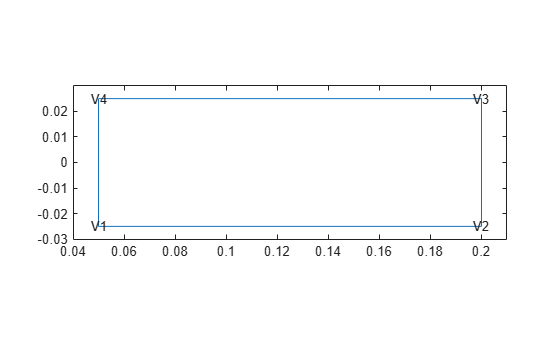Since R2023a

## Description

A `vertexLoad` object contains a description of a load on a vertex of a geometry. An `femodel` object contains an array of `vertexLoad` objects in its `VertexLoad` property.

## Creation

### Syntax

``model.VertexLoad(VertexID) = vertexLoad(Force=Value)``

### Description

example

````model.VertexLoad(VertexID) = vertexLoad(Force=Value)` creates a `vertexLoad` object and sets its Force property using a name-value argument. This syntax assigns the specified concentrated force to the specified vertices of the geometry stored in the `femodel` object `model`. For example, ```model.VertexLoad([1 2]) = vertexLoad(Force=[0 1000 0])``` specifies the concentrated force on vertices 1 and 2.You can specify force for a static, transient, or frequency response structural analysis. A structural modal analysis cannot have concentrated force.```

### Input Arguments

expand all

Vertex IDs, specified as a vector of positive integers. Find the vertex IDs using `pdegplot` with the `VertexLabels` value set to `"on"`.

Data Types: `double`

## Properties

expand all

Concentrated force at a vertex, specified as a vector or function handle. Use a function handle to specify concentrated force that depends on time or frequency. For details, see Nonconstant Parameters of Finite Element Model.

Data Types: `double` | `function_handle`

## Examples

collapse all

Specify a force value at a vertex for an `femodel` object representing a static structural problem.

Create and plot a rectangular geometry.

```gm = decsg([3 4 0.05 0.2 0.2 0.05 -0.025 -0.025 0.025 0.025]'); pdegplot(gm,VertexLabels="on"); xlim([0.04 0.21]) ylim([-0.03 0.03])```Create an `femodel` object for solving a static structural problem, and assign the geometry to the model.

```model = femodel(AnalysisType="structuralStatic", ... Geometry=gm);```

Specify the concentrated force at vertex 1.

```model.VertexLoad(1) = vertexLoad(Force=[0 10^4]); model.VertexLoad```
```ans = 1x4 vertexLoad array Properties for analysis type: structuralStatic Index Force 1 [0 10000] 2 [] 3 [] 4 [] Show all properties ```

## Version History

Introduced in R2023a